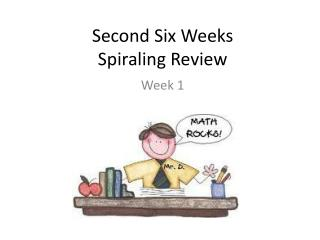DownloadDownload PresentationSecond Six Weeks Spiraling Review

Second Six Weeks Spiraling Review

Télécharger la présentationSecond Six Weeks Spiraling Review

- - - - - - - - - - - - - - - - - - - - - - - - - - - E N D - - - - - - - - - - - - - - - - - - - - - - - - - - -
Presentation Transcript

1. Day 1 76, 435 • Write the following number in expanded notation and in words. • If you wanted to change the number to 76,415, what would you do? • Write a number sentence to show the operation needed to make the change.

2. Day 2 FACT TIME!!!!

3. Day 3 • Order the following numbers from least to greatest vertically. • What patterns do you notice? 6,422 7,533 5,311

4. Day 4 Use base ten blocks. Rylan had 55 cars. He gave some of his cars to his brother, Justin. Now Rylan has 39 cars. How many cars did Rylan give his brother? • What do you need to find out? • What operation will you use and why? • Write a number sentence to show how you solved the problem. Label your answer.

5. Day 5 FACT TIME!!!!

6. Day 1 Use base ten blocks. Martin collects sea shells. He shared 24 with Marcy. Now Martin has 48 sea shells. How many sea shells did Martin have before he shared with Marcy? • What do you need to find out? • What operation will you use and why? • Write a number sentence to show how you solved the problem. Label your answer.

7. Day 2 FACT TIME!!!!

8. Day 3 • Write the following number in expanded notation and in words: 265,030 B) To make the number 245,031, what places would be changed? C) Explain how you would solve the problem and what operation(s) you would use.

9. Day 4 0 9 1 2 7 1 • What is the greatest 6-digit number that can be created? • Write the 6-digit number with the least possible value. • Write each number you created in expanded notation.

10. Day 5 FACT TIME!!!!

11. Day 1 Use base ten blocks. Solve the following riddle. If you added 3 tens and subtracted 9 ones to the magic number, you would have 901. • What is the magic number? • Explain how you solved the problem.

12. Day 2 FACT TIME!!!!

13. Day 3 Use base ten blocks. Juanita had 97 glass ornaments. Yesterday she tripped over her 23 pound dog and broke a dozen ornaments. How many ornaments does Juanita Have? • What operation(s) will you use and why? • Show how you solved the problem. Label your answer.

14. Day 4 7 0 8 8 • What is the greatest two-digit number that can be created using the numbers above? • What is the least three-digit number that can be created using the numbers above? • Write a number sentence to show the difference between the two created numbers.

15. Day 5 FACT TIME!!!!

16. Day 1 Solve the following riddle. If you added 2 hundred and subtracted 4 ones from the magic number, you would have 641. • What is the magic number? • Write a number sentence to show how you solved the problem.

17. Day 2 FACT TIME!!!!

18. Day 3 Look at the following number: 47,206 • Which digit is in the hundreds place? • Which digit is in the ten-thousands place? • Write the number in expanded notation.

19. Day 4 Write a comparison sentence for each of the following using symbols (<, =, >). Name the place that helped you compare the numbers. Example: 6,943 > 6,934 • 8,730 8,783 • 5,001 4,999 • 6,346 6,346 • 2,116 2,016

20. Day 5 FACT TIME!!!!

21. Day 1 Use counters to solve the problem. Parker wants to buy as many toys as she can with her ten dollar bill. She can buy individual ponies for \$2 each or a set of 2 dolls for \$3. What should she buy and why? • What do you need to find out? • What operation(s) will you use and why? • Write a number sentence to show you how you solved the problem. Label your answer.

22. Day 2 FACT TIME!!!!

23. Day 3 Look at the groups of triangles below: If the pattern stays the same, how many triangles will be in the next group? Draw a picture to show how you know. Describe the change in the number of triangles from one group to the next.

24. Day 4 6,004 6,080 6,099 6,082 • Write these numbers in order from least to greatest. • What places did you use to write the numbers in order?

25. Day 5 FACT TIME!!!!